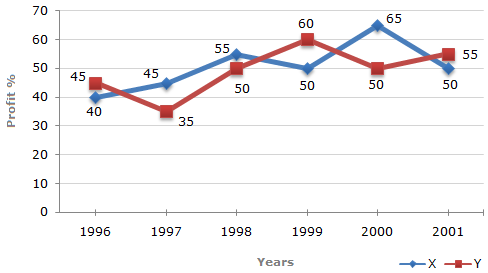# Data Interpretation - Line Charts - Discussion

Discussion Forum : Line Charts - Line Chart 4 (Q.No. 4)
Directions to Solve

The following line graph gives the percent profit earned by two Companies X and Y during the period 1996 - 2001.

Percentage profit earned by Two Companies X and Y over the Given Years

 %Profit = Income - Expenditure x 100 Expenditure4.
The expenditure of Company X in the year 1998 was Rs. 200 crores and the income of company X in 1998 was the same as its expenditure in 2001. The income of Company X in 2001 was ?
Rs. 465 crores
Rs. 385 crores
Rs. 335 crores
Rs. 295 crores
Explanation:

Let the income of Company X in 1998 be Rs. x crores.

 Then, 55 = x - 200 x 100x = 310. 200Expenditure of Company X in 2001 = Income of Company X in 1998 = Rs. 310 crores.

Let the income of Company X in 2001 be Rs. z crores.

 Then, 50 = z - 310 x 100z = 465. 310Income of Company X in 2001 = Rs. 465 crores.

Discussion:
6 comments Page 1 of 1.

Jamyang Tenzin said:   2 years ago
How comes 310? please give a clear explanation.

Deepika said:   7 years ago
@Sandeep.

150 is the profit % of X in 2001.

Sandeep said:   7 years ago
How did 150 come?

Vignes said:   7 years ago
Company (x) 1998.

155%of200 = 310.

Income (x) 1998 = Expenditure (x) 2001.

Company (x) 2001.

150%of310 = 465.

Akash said:   7 years ago

50 = z - 310 / 310 * 100.

Gyandeep Hota said:   1 decade ago
SHORT CUT:
NOTE:

Always Expenditure is 100% and .
Income =Expenditure + profit.

Solution:

Given that Expenditure(x)1998 = 200cr.
Income(x)1998 = Expenditure(x)2001.
Find Income(x)2001=?

155 = x(let income)[155 means expenditure+profit i.e 100+55).

100 =b200(given).

Then (200*155)/100 = x.

x = 310 = Expenditure(x)2001 (given).

Again,

150 = y (let).

100 = 310.

y = (150*310)/100.